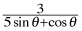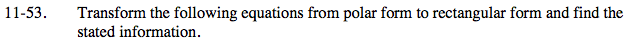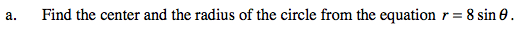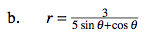Home > PC > Chapter 11 > Lesson 11.1.4 > Problem11-53

11-53.
1. Transform the following equations from polar form to rectangular form and find the stated information. Homework Help ✎

1. Find the center and the radius of the circle from the equation r = 8 sin θ.

2. r =$r^2=8r\sin{\theta}$

x2 + y2 = 8y

Complete the square so that you can identify the center and radius of the circle.$5r\sin{\theta}+r\cos\theta=3$

5y + x = 3